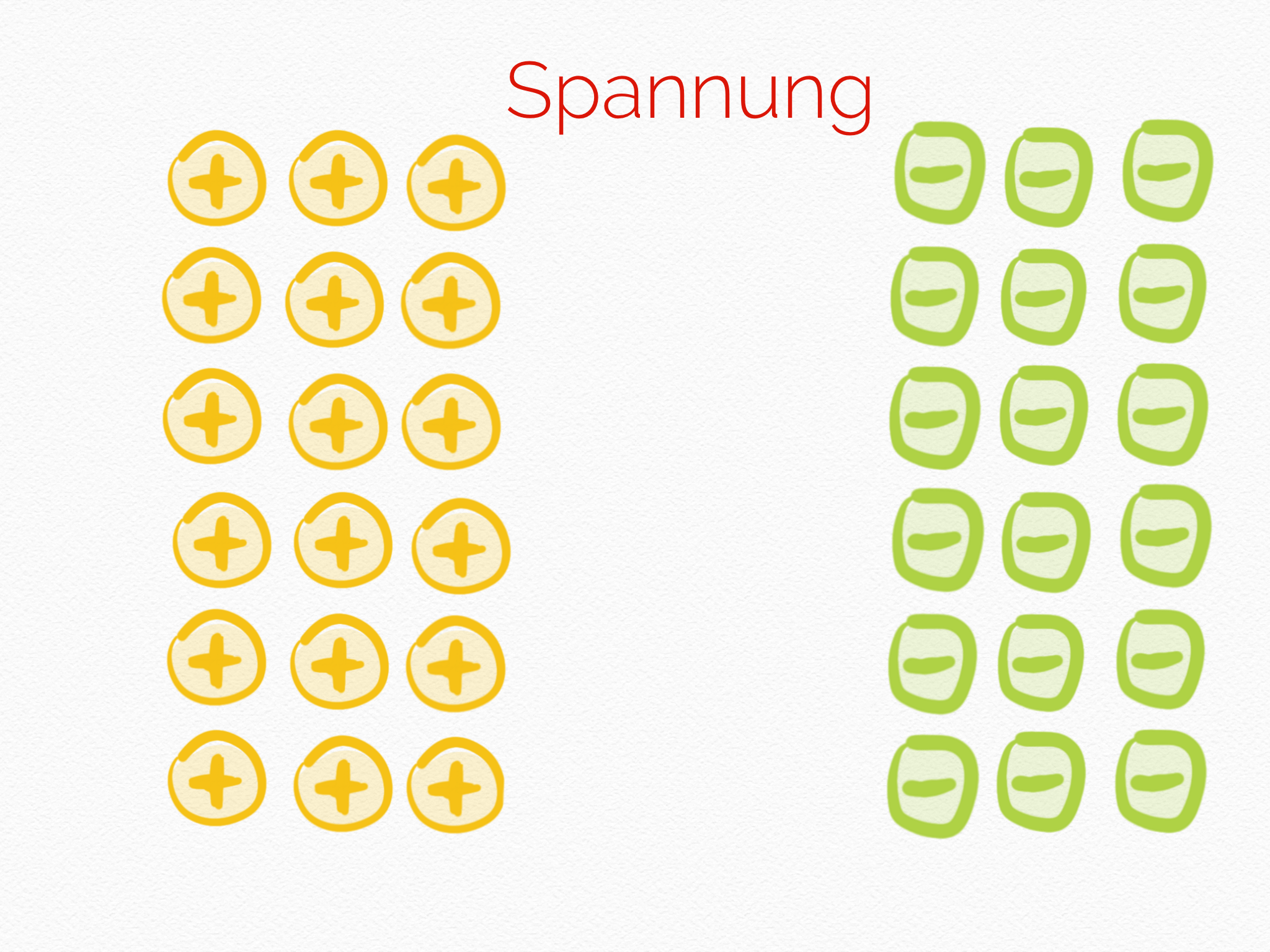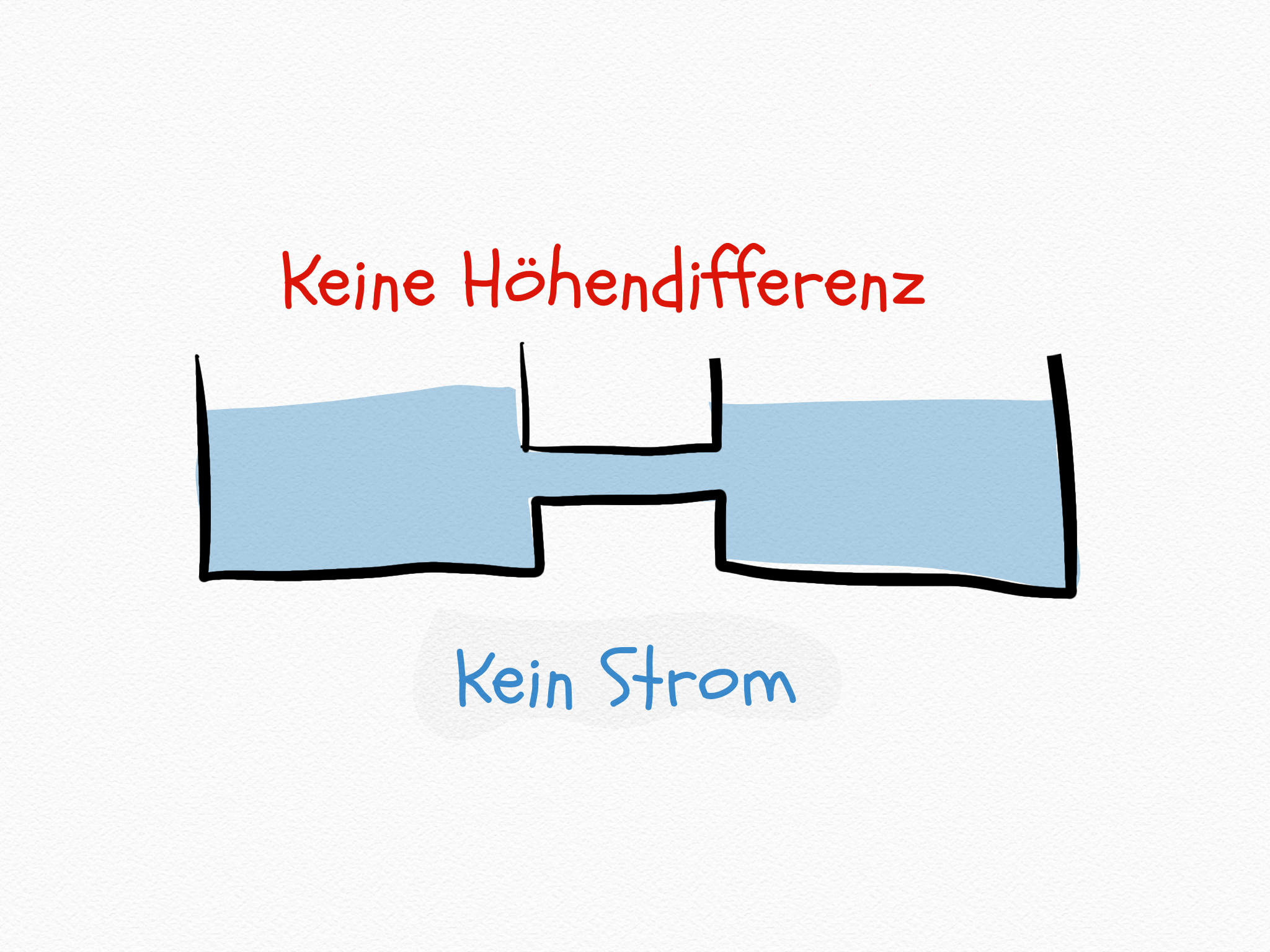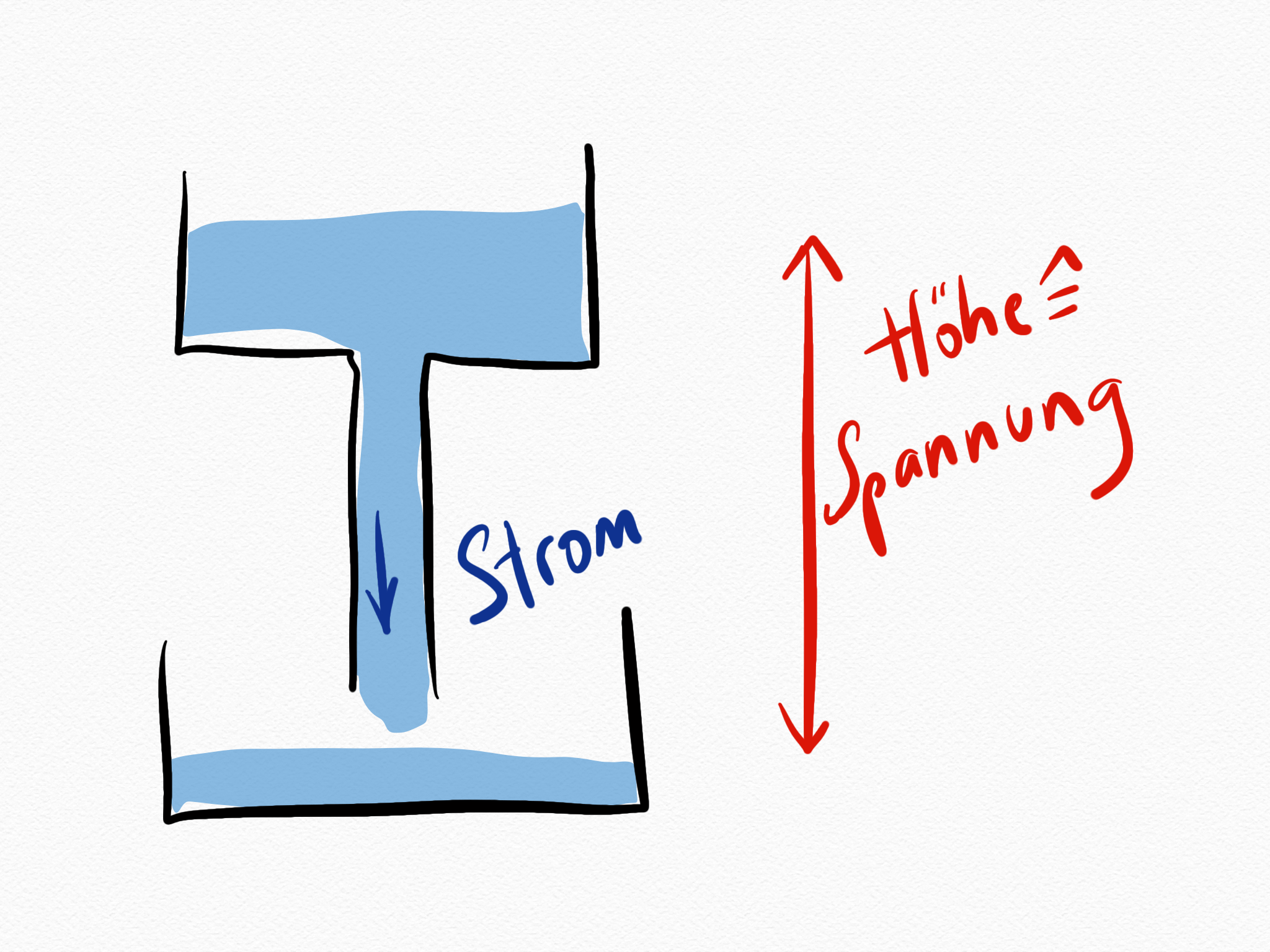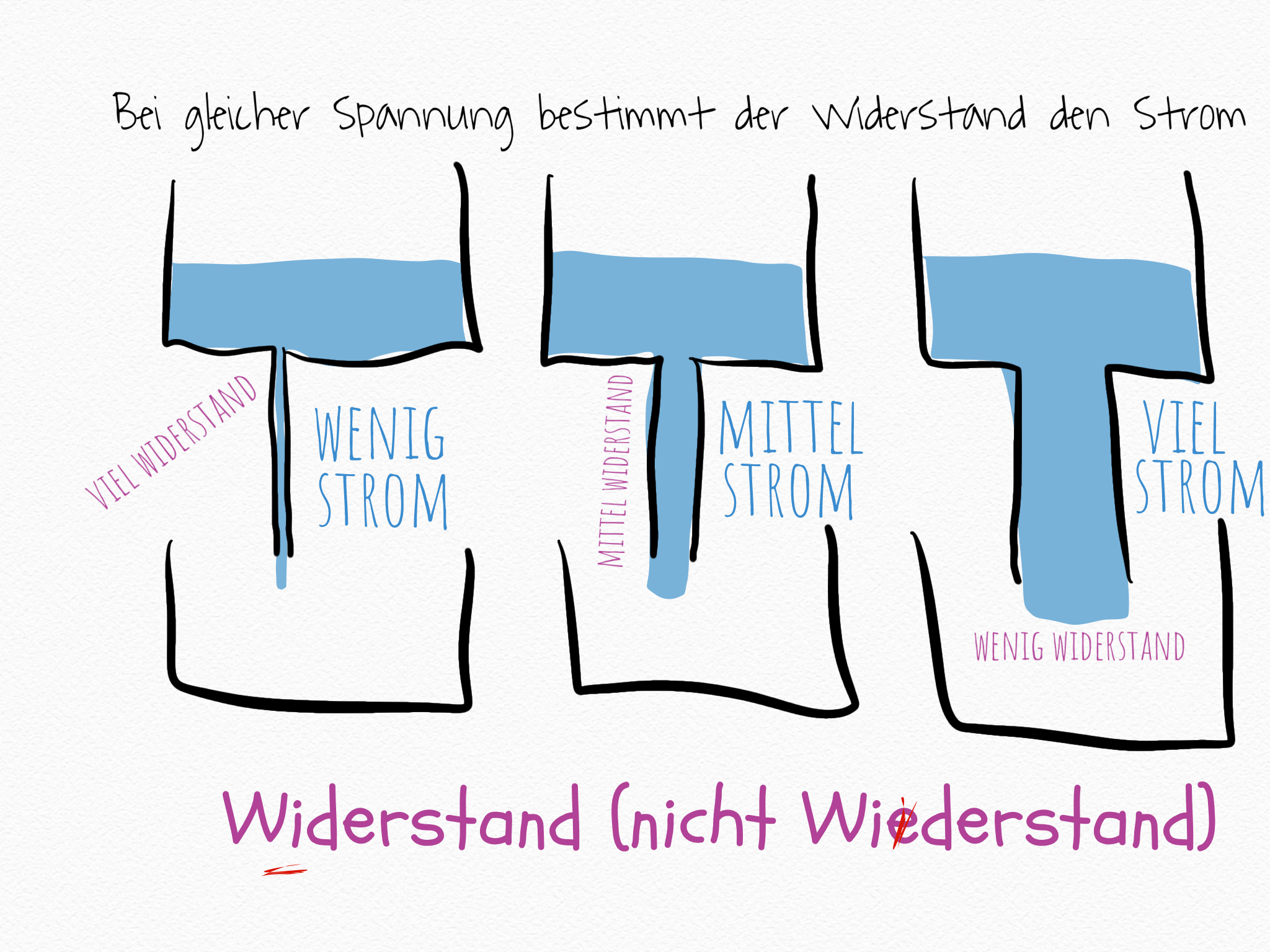# Can current be applied more than voltage

## What is the difference between voltage and current?

Electric voltage arises when charges are present separately. On the one hand positive charges +++ and on the other hand negative charges - - -

Electric current is created when there is voltage. And when the electrical charges can equalize because there is an electrical conductor. Electric current is the flow of electrically charged particles.

The electrical resistance determines how much current can flow at a given voltage.

Wikipedia keywords: electricity

1. The unit of the electrical voltage U is 1 volt.
2. The unit of the electric current I is 1 ampere.
3. The unit of electrical resistance is 1 ohm.### Describe

Voltage is a prerequisite for current flow. Somewhere there are more negative electrical charges than positive ones. This creates a potential difference that is referred to as voltage. A conductive connection between the areas of different potential is a second prerequisite. Free charge carriers are a third requirement for electricity to flow.

### to compare

You can compare this to two pools of water that are at different heights. The water in the higher pool has a higher potential energy than the water in the lower pool. The difference makes the flow of water possible if there is a suitable connection between the two basins. It does not matter what absolute height the two pools are at - only their height difference is decisive.Even with the electrical voltage, it does not matter how many charges are absolutely present where, it is decisive that more or less of them are present elsewhere.

The comparison also shows that electricity is not used. The water is also not lost by flowing. So are the electrical charges. What is consumed, however, is the difference in height of the water - is the electrical voltage when electricity flows. The battery is "running out".

### Expand

"Ohm's law" states how current, voltage and resistance are related. The more voltage, the more current. The more resistance, the less current.

The following applies: U = R • I### Still interested?

1. The program with the mouse. Well explained.

1. DC or AC? The fantastic inventor Nikola Tesla. Link to listen.